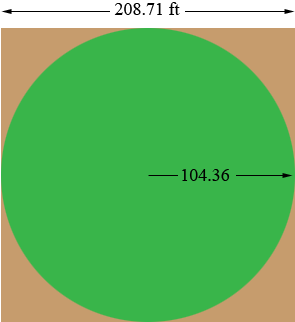SEARCH HOMEMath Central Quandaries & QueriesQuestion from Scott, a student: I'm trying to find out the sq footage of the corners of an acre. If an acre is 43,560 sq and if I have this right the surface area of the circumference is 1040 ft. what is the combined square footage of the 4 corners? Or the percentage the original acre?Scott,

I don't understand what you are asking. Do you have a circle inside a square where the square has area an acre and you want the area in the corners outside the circle?

Harley

Scott wrote back

Yes that's it. Sorry I didn't explain it better.

Scott,

An acre is 43,560 square feet so if you have a square with area one acre and each side is x feet long then

x2 = 43,560

so

x = √43,560 = 208.71 feet.

Hence the radius of the inscribed circle is 208.71/2 = 104.36 feet.What is the area of a circle with radius 104.36 feet? Subtract this from 43,560 square feet to find the area of the corner pieces.

HarleyMath Central is supported by the University of Regina and The Pacific Institute for the Mathematical Sciences.